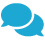Complete Solution for Both Individuals and Businesses. Hire Us021-35873744

Monthly Yearly
Income 0.00 0.00
Tax 0.00 0.00
Net 0.00 0.00

# Income Tax Slabs 2023

As per income tax exemption bill passed by Government of Pakistan, following slabs and income tax rates will be applicable for salaried persons for the year 2022-2023

• Where the taxable income income does not exceed Rs. 600,000 the rate of income tax is 0%.
• Where the taxable income income exceeds Rs. 600,000 but does not exceed Rs. 1,200,000 the rate of income tax is 2.5% of the amount exceeding Rs. 600,000.
• Where the taxable income income exceeds Rs. 1,200,000 but does not exceed Rs. 2,400,000 the rate of income tax is Rs. 15,000 + 12.5% of the amount exceeding Rs. 1,200,000.
• Where the taxable income income exceeds Rs. 2,400,000 but does not exceed Rs. 3,600,000 the rate of income tax is Rs. 165,000 + 12.5% of the amount exceeding Rs. 2,400,000.
• Where the taxable income income exceeds Rs. 3,600,000 but does not exceed Rs. 6,000,000 the rate of income tax is Rs. 405,000 + 17.5% of the amount exceeding Rs. 3,600,000.
• Where the taxable income income exceeds Rs. 6,000,000 but does not exceed Rs. 12,000,000 the rate of income tax is Rs. 1005000 + 32.5% of the amount exceeding Rs. 6,000,000.
• Where the taxable income income exceeds Rs. 12,000,000 the rate of income tax is Rs. 2,955,000 + 35% of the amount exceeding Rs. 12,000,000.

Note: The above calculation tool is correct to the best of our knowledge, but it may transfigure or change with the passage of time. We cannot guarantee the correctness in calculation. We do not accept responsibility for any loss or expense in any case resulting from the use of this tax calculator. For more details visit FBR website

Monthly Yearly
Income 0.00 0.00
Tax 0.00 0.00
Net 0.00 0.00

# Income Tax Slabs 2022

As per income tax exemption bill passed by Government of Pakistan, following slabs and income tax rates will be applicable for salaried persons for the year 2021-2022

• Where the taxable salary income does not exceed Rs. 600,000 the rate of income tax is 0%.
• Where the taxable salary income exceeds Rs. 600,000 but does not exceed Rs. 1,200,000 the rate of income tax is 5% of the amount exceeding Rs. 600,000.
• Where the taxable salary income exceeds Rs. 1,200,000 but does not exceed Rs. 1,800,000 the rate of income tax is Rs. 30,000 + 10% of the amount exceeding Rs. 1,200,000.
• Where the taxable salary income exceeds Rs. 1,800,000 but does not exceed Rs. 2,500,000 the rate of income tax is Rs. 90,000 + 15% of the amount exceeding Rs. 1,800,000.
• Where the taxable salary income exceeds Rs. 2,500,000 but does not exceed Rs. 3,500,000 the rate of income tax is Rs. 195,000 + 17.5% of the amount exceeding Rs. 2,500,000.
• Where the taxable salary income exceeds Rs. 3,500,000 but does not exceed Rs. 5,000,000 the rate of income tax is Rs. 370,000 + 20% of the amount exceeding Rs. 3,500,000.
• Where the taxable salary income exceeds Rs. 5,000,000 but does not exceed Rs. 8,000,000 the rate of income tax is Rs. 670,000 + 22.5% of the amount exceeding Rs. 5,000,000.
• Where the taxable salary income exceeds Rs. 8,000,000 but does not exceed Rs. 12,000,000 the rate of income tax is Rs. 1,345,000 + 25% of the amount exceeding Rs. 8,000,000.
• Where the taxable salary income exceeds Rs. 12,000,000 but does not exceed Rs. 30,000,000 the rate of income tax is Rs. 2,345,000 + 27.5% of the amount exceeding Rs. 12,000,000.
• Where the taxable salary income exceeds Rs. 30,000,000 but does not exceed Rs. 50,000,000 the rate of income tax is Rs. 7,295,000 + 30% of the amount exceeding Rs. 30,000,000.
• Where the taxable salary income exceeds Rs. 50,000,000 but does not exceed Rs. 75,000,000 the rate of income tax is Rs. 13,295,000 + 32.5% of the amount exceeding Rs. 50,000,000.
• Where the taxable salary income exceeds Rs. 75,000,000 the rate of income tax is Rs. 21,420,000 + 35% of the amount exceeding Rs. 75,000,000.

Note: The above calculation tool is correct to the best of our knowledge, but it may transfigure or change with the passage of time. We cannot guarantee the correctness in calculation. We do not accept responsibility for any loss or expense in any case resulting from the use of this tax calculator. For more details visit FBR website